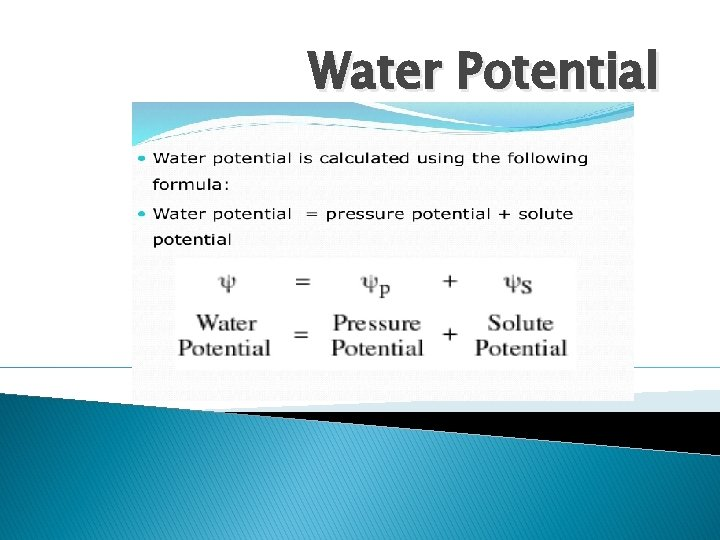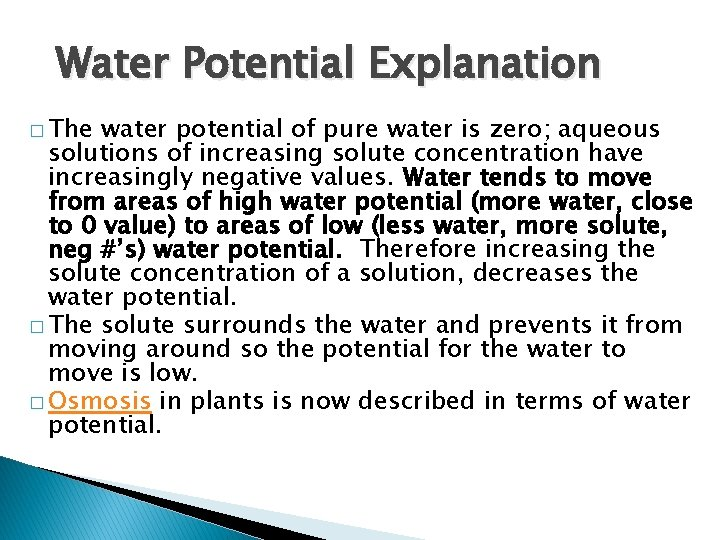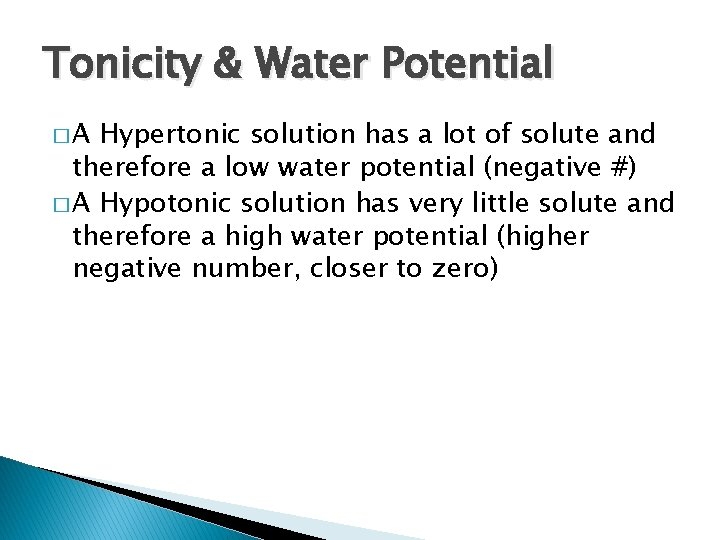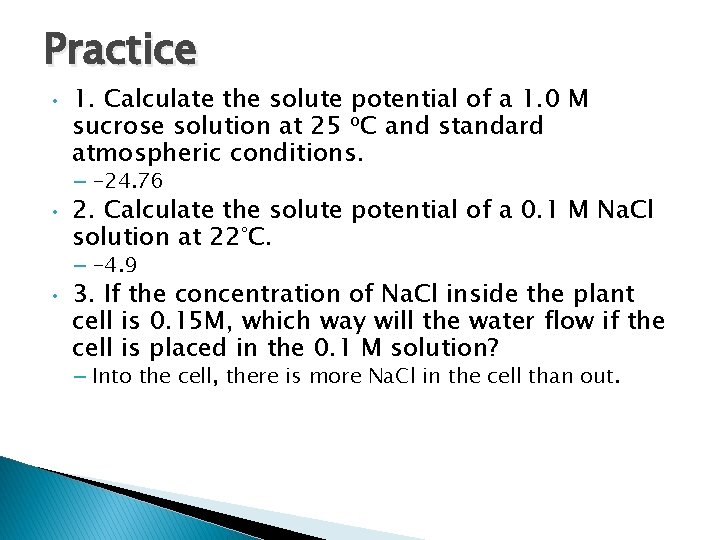# Water Potential Water Potential Explanation The water potential

• Slides: 6Water PotentialWater Potential Explanation � The water potential of pure water is zero; aqueous solutions of increasing solute concentration have increasingly negative values. Water tends to move from areas of high water potential (more water, close to 0 value) to areas of low (less water, more solute, neg #’s) water potential. Therefore increasing the solute concentration of a solution, decreases the water potential. � The solute surrounds the water and prevents it from moving around so the potential for the water to move is low. � Osmosis in plants is now described in terms of water potential.Ψ = Ψ S + ΨP • • (Ψs) If the solute concentration of a solution increases, the potential for the water in that solution to undergo osmosis decreases. (Ψs) Therefore, the more solute that is added to a solution, the more negative its osmotic (solute) potential gets. (ΨP). If no physical pressure is applied to a solution, then the solute potential is equal to the water potential. (ΨP). However, if physical pressure is applied to a solution, then it’s water potential (the potential for the water to move and do work) will be affected.Solute potential formula � Solute potential (Ψs) = –i. CRT � i = The number of particles the molecule will make in water; for Sucrose this is given as 1 , for Na. Cl, it’s 2 since Na. Cl forms 2 ions, Na+ and Cl� C = Molar concentration (from your experimental data) � R = Pressure constant = 0. 0831 liter bar/mole K � T = Temperature in degrees Kelvin = 273 + °C of solutionTonicity & Water Potential �A Hypertonic solution has a lot of solute and therefore a low water potential (negative #) � A Hypotonic solution has very little solute and therefore a high water potential (higher negative number, closer to zero)Practice • 1. Calculate the solute potential of a 1. 0 M sucrose solution at 25 o. C and standard atmospheric conditions. – -24. 76 • 2. Calculate the solute potential of a 0. 1 M Na. Cl solution at 22°C. – -4. 9 • 3. If the concentration of Na. Cl inside the plant cell is 0. 15 M, which way will the water flow if the cell is placed in the 0. 1 M solution? – Into the cell, there is more Na. Cl in the cell than out.